Examples

Chapter 16 Class 11 Probability (Term 2)
Serial order wise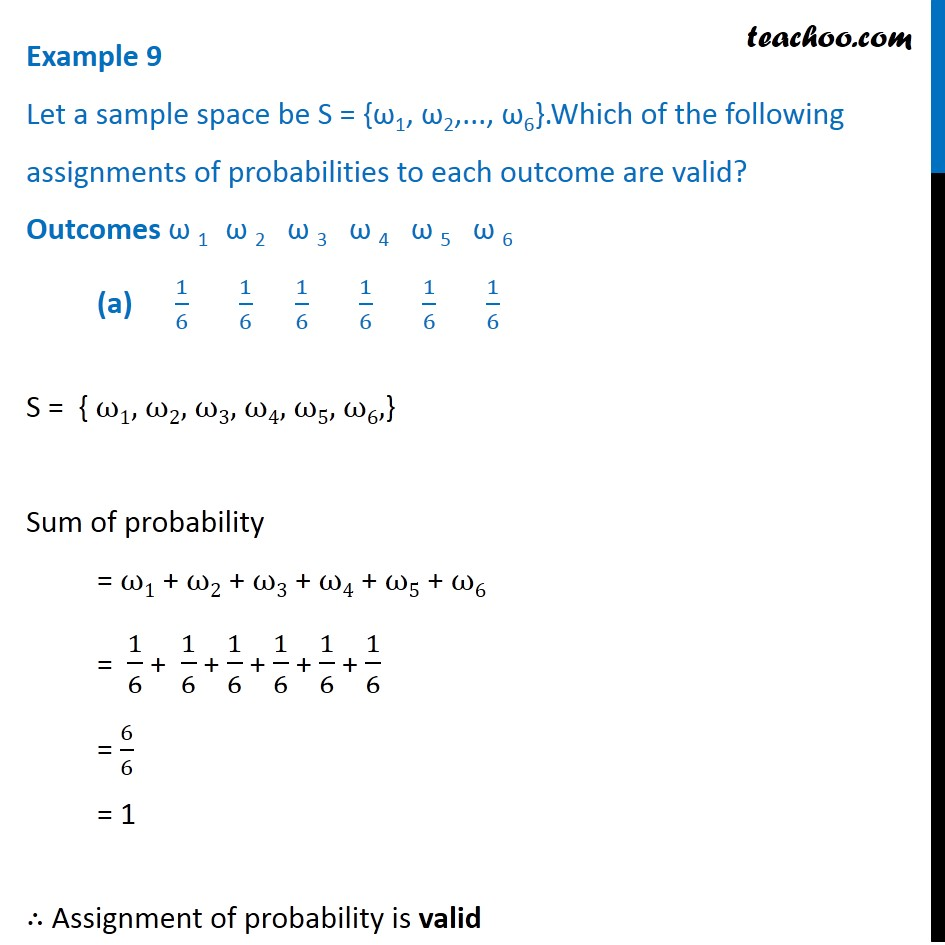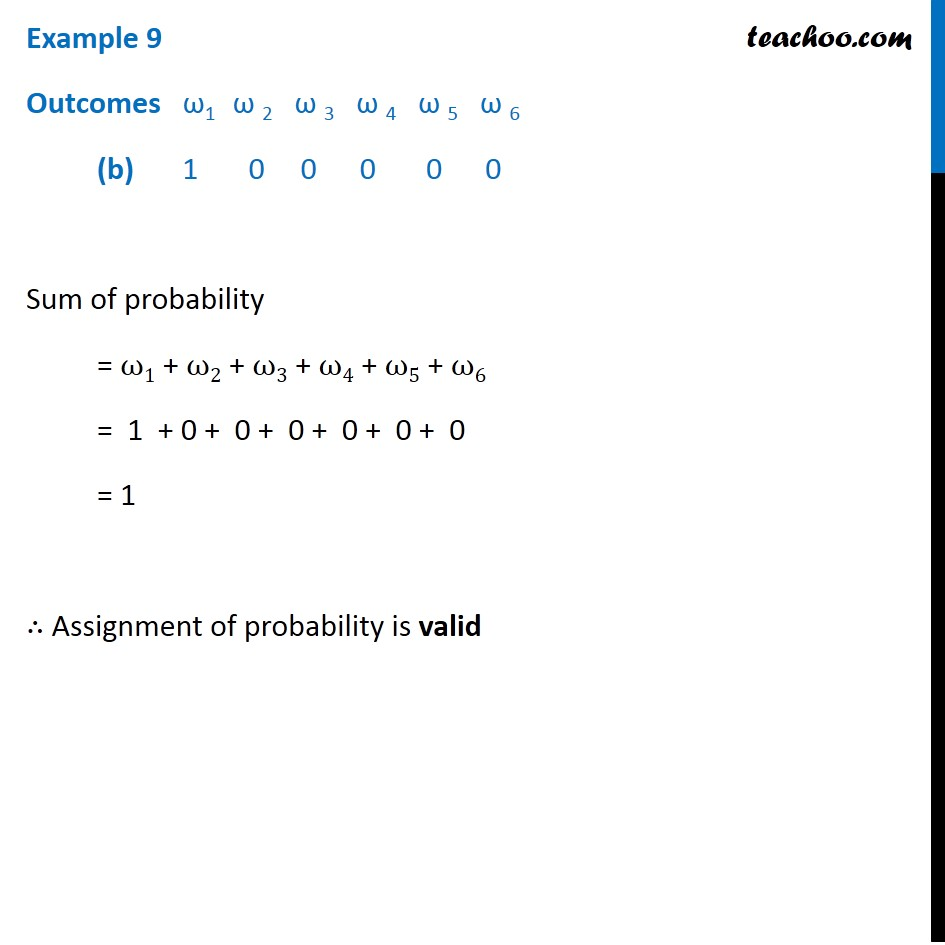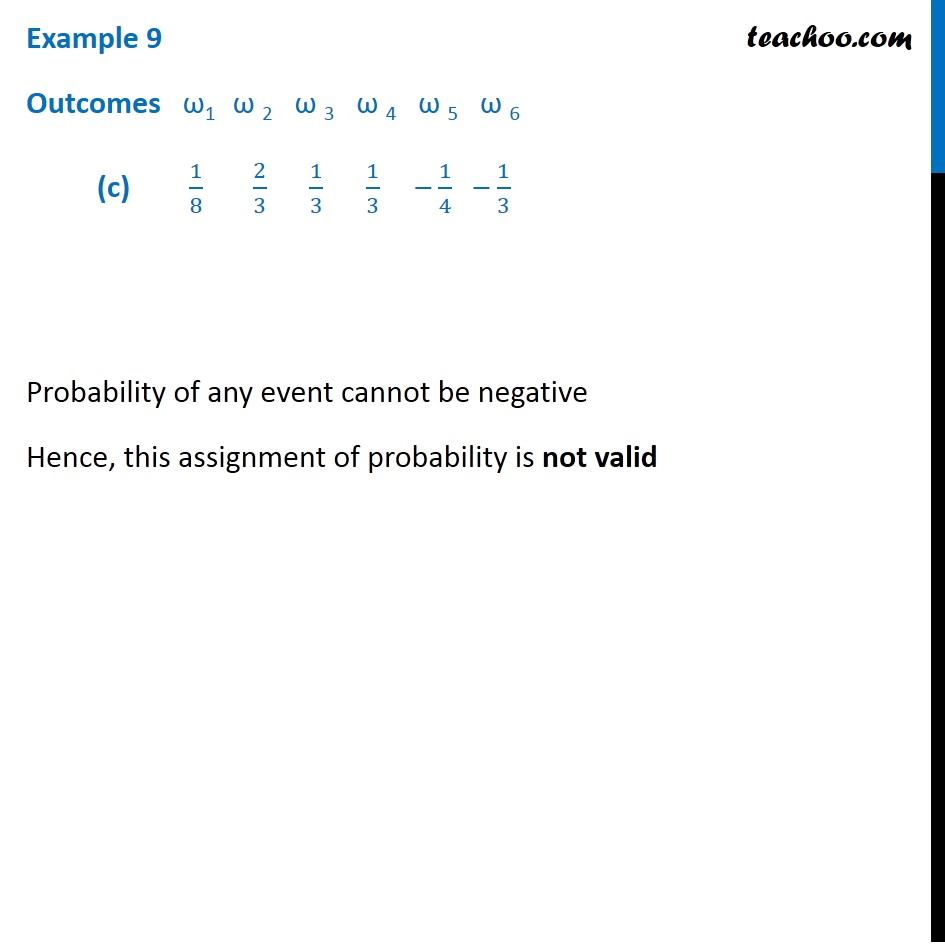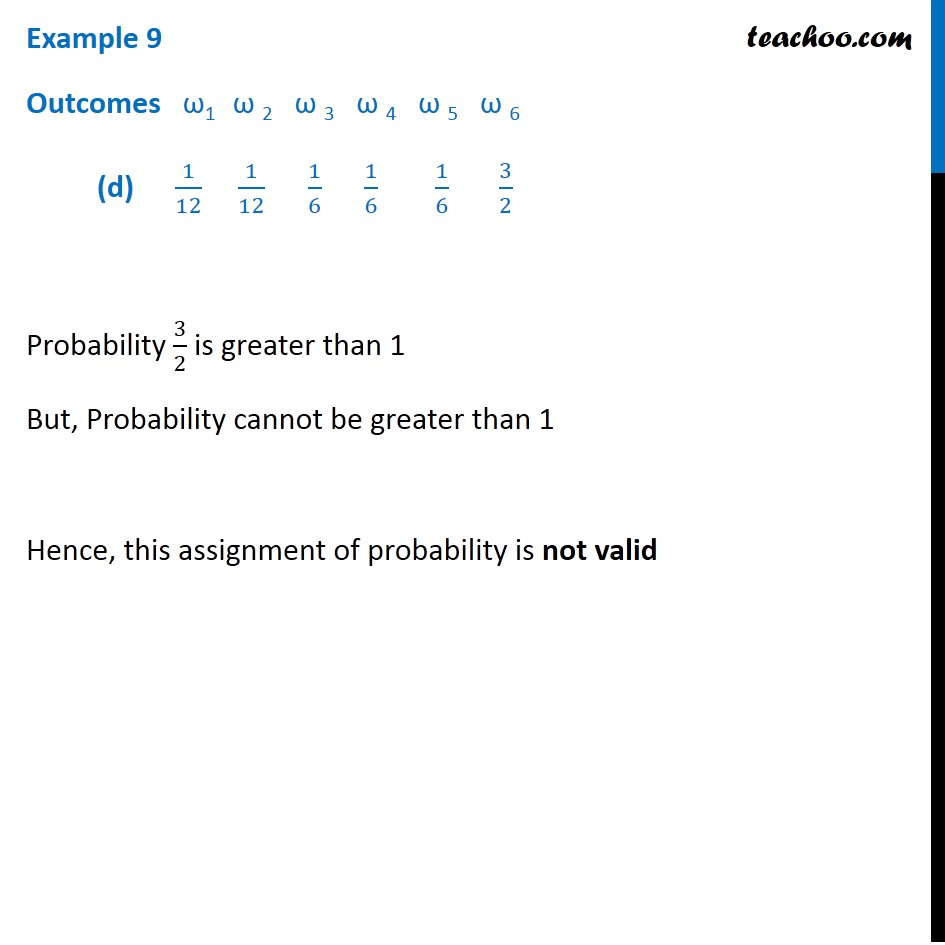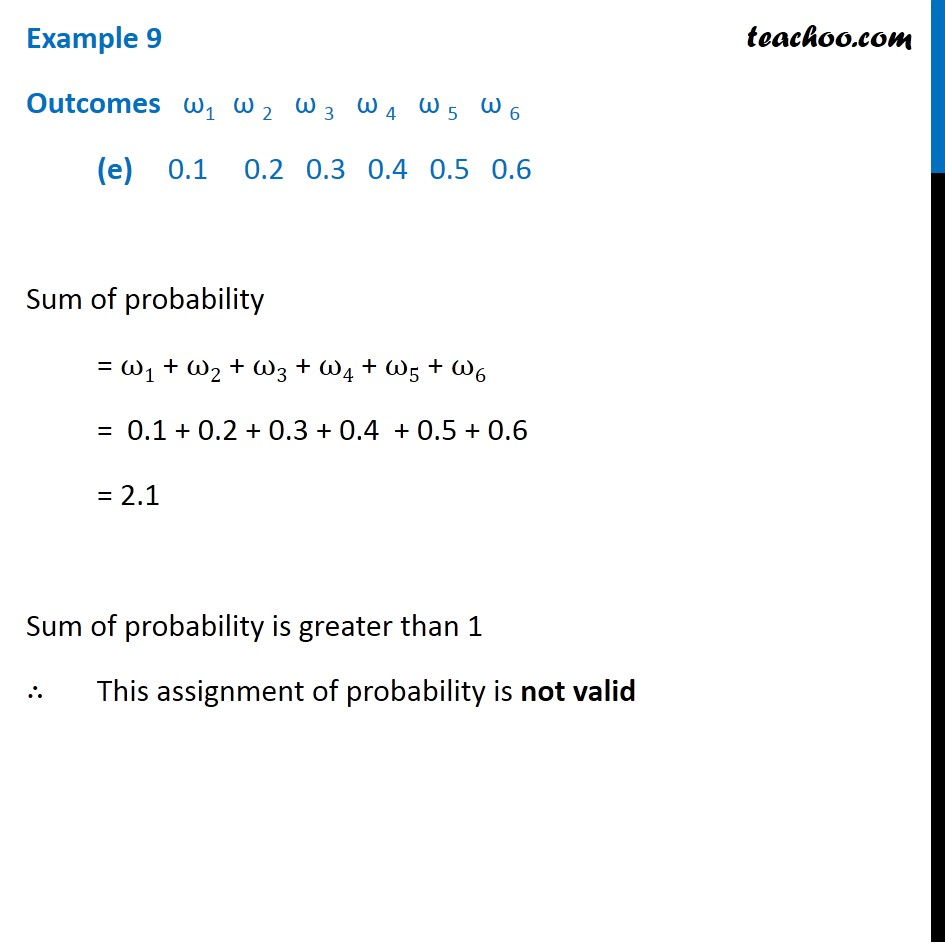641 students have Teachoo Black. What are you waiting for?

### Transcript

Example 9 Let a sample space be S = {ω1, ω2,..., ω6}.Which of the following assignments of probabilities to each outcome are valid? Outcomes ω 1 ω 2 ω 3 ω 4 ω 5 ω 6 (a) 1/6 1/6 1/6 1/6 1/6 1/6 S = { ω1, ω2, ω3, ω4, ω5, ω6,} Sum of probability = ω1 + ω2 + ω3 + ω4 + ω5 + ω6 = 1/6 + 1/6 + 1/6 + 1/6 + 1/6 + 1/6 = 6/6 = 1 ∴ Assignment of probability is valid Example 9 Outcomes ω1 ω 2 ω 3 ω 4 ω 5 ω 6 (b) 1 0 0 0 0 0 \ Sum of probability = ω1 + ω2 + ω3 + ω4 + ω5 + ω6 = 1 + 0 + 0 + 0 + 0 + 0 + 0 = 1 ∴ Assignment of probability is valid Example 9 Outcomes ω1 ω 2 ω 3 ω 4 ω 5 ω 6 (c) 1/8 2/3 1/3 1/3 – 1/4 – 1/3 Probability of any event cannot be negative Hence, this assignment of probability is not valid Example 9 Outcomes ω1 ω 2 ω 3 ω 4 ω 5 ω 6 (d) 1/12 1/12 1/6 1/6 1/6 3/2 Probability 3/2 is greater than 1 But, Probability cannot be greater than 1 Hence, this assignment of probability is not valid Example 9 Outcomes ω1 ω 2 ω 3 ω 4 ω 5 ω 6 (e) 0.1 0.2 0.3 0.4 0.5 0.6 Sum of probability = ω1 + ω2 + ω3 + ω4 + ω5 + ω6 = 0.1 + 0.2 + 0.3 + 0.4 + 0.5 + 0.6 = 2.1 Sum of probability is greater than 1 ∴ This assignment of probability is not valid